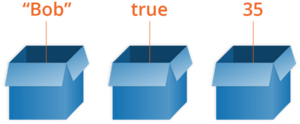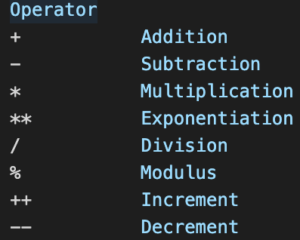# Java Basics Tutorial – Part 4 – Data and Calculations

In this part of the Java Bascics Tutorial, we take a look at Data and Calculations.Data types specify the different sizes and values stored in a variable. There are two types of data in Java:

• Primitive data types
• Non-primitive data types

To start, we will be using these data types only:• int –  holds a wide range of non-fractional number values.
• double – only takes up to 8 bits of memory
• boolean – can contain only two values: true or false.
• char – is a symbol representing a Unicode-encoded character.
• String – is a sequence of charactersBesides printing them on the console, we can use them for various arithmetic operations. The table on the right shows different types of arithmetic operations. All of them are the same as the arithmetic operations you have learned in school.

These may seem like simple operators, but when correctly, used they can be of help on more complex projects.

## Lesson Topics

In this video we review the following topics:
• Variables
• Data Types
• Statements
• Arithmetic Operators
• Expressions

## Practical Exercises

Watch the video and solve the problemsTo better understand the material, do the coding exercises and implement the knowledge you acquired. Writing code is the only way to master the skill of code.

Submit your code in the SoftUni Judge System: